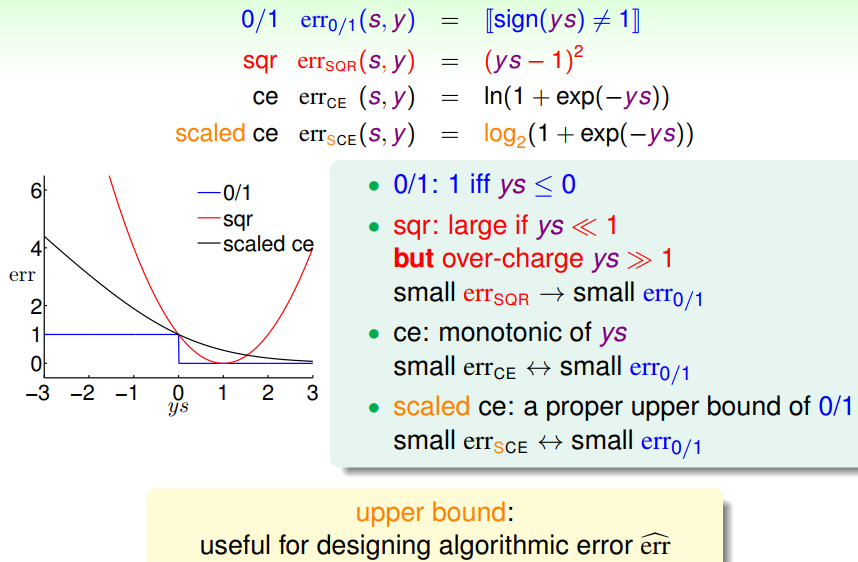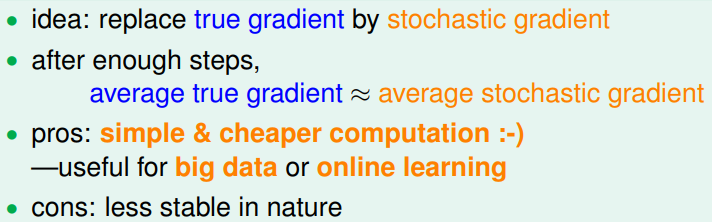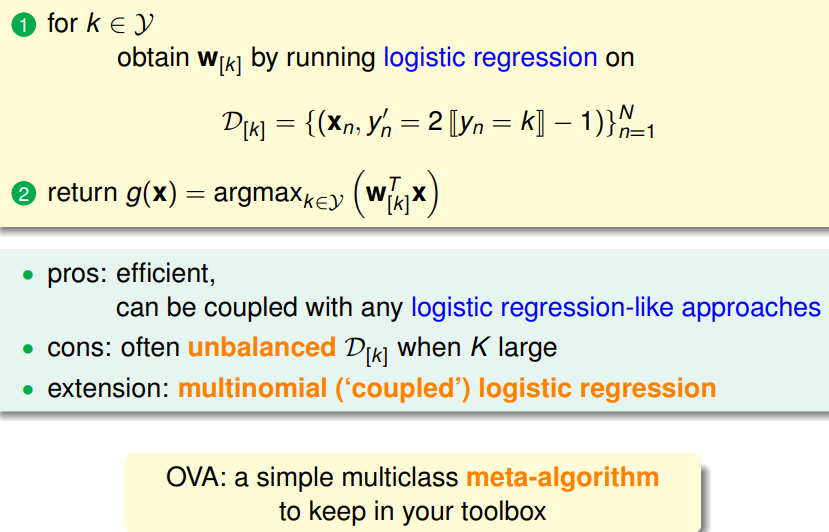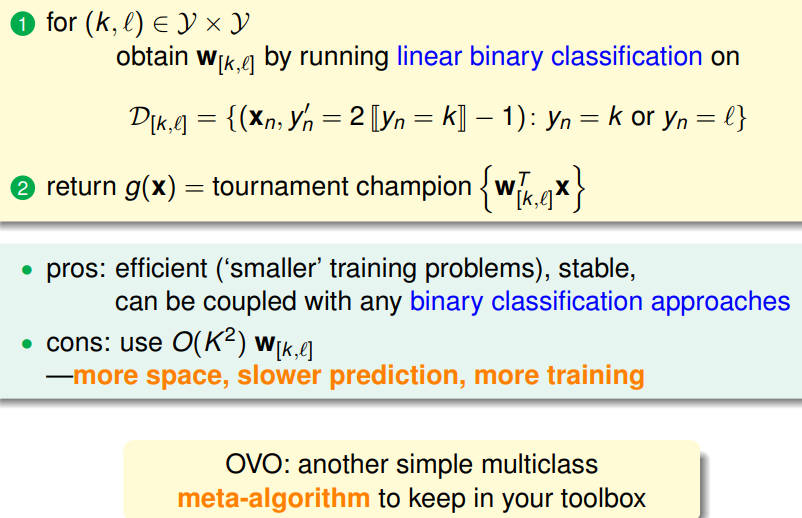# 机器学习基石 - Linear Models for Classification

Hsuan-Tien Lin, 林轩田，副教授 (Associate Professor)，资讯工程学系 (Computer Science and Information Engineering)

## Linear Models for Binary Classification

• $ys$$ys$: classification correctness score 分类正确性分数

• Visualizing Error Functions• $small\ E^{CE}_{in} (\mathbf w) \Rightarrow small\ E_{out}^{0/1}(\mathbf w)$

logistic/linear reg. for linear classification

• linear regression sometimes used to set ${\mathbf{w}}_{0}$$\mathbf w_0$ for PLA/pocket/logistic regression

• logistic regression often preferred over pocket• two practical rule-of-thumb:
• stopping condition? t large enough
• $\eta$$η$? 0.1 when x in proper range

## Multiclass via Logistic Regression

• One Class at a Time Softly 属于某个类别的概率

• One-Versus-All (OVA) Decomposition## Multiclass via Binary Classification

• One-versus-one (OVO) Decompositionvoting of classifiers